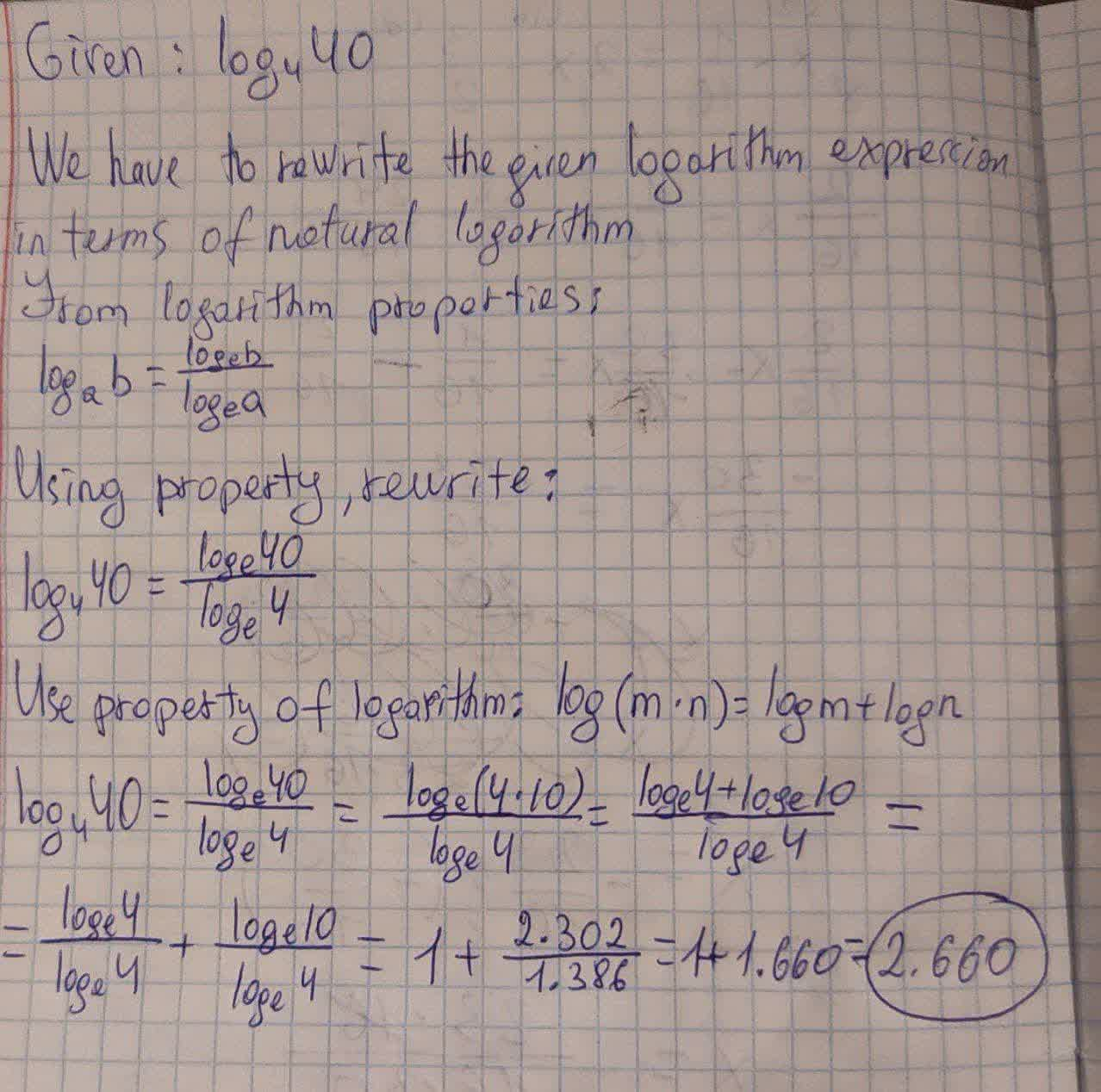# Write the following logarithms in terms of the natural logarithm.Nannie Mack 2021-08-17 Answered
Write the following logarithms in terms of the natural logarithm. Then use a calculator to find the value of the logarithm, rounding your result to four decimal places.
$$\displaystyle{{\log}_{{{4}}}{\left\lbrace{40}\right\rbrace}}$$

• Questions are typically answered in as fast as 30 minutes

### Plainmath recommends

• Get a detailed answer even on the hardest topics.
• Ask an expert for a step-by-step guidance to learn to do it yourself.toroztatG
The logarithm will be: